The AUTOREG Procedure

## Example 8.1 Analysis of Real Output Series

In this example, the annual real output series is analyzed over the period 1901 to 1983 (Balke and Gordon; 1986, pp. 581–583). With the following DATA step, the original data are transformed using the natural logarithm, and the differenced series DY is created for further analysis. The log of real output is plotted in Output 8.1.1.

```title 'Analysis of Real GNP';
data gnp;
date = intnx( 'year', '01jan1901'd, _n_-1 );
format date year4.;
input x @@;
y  = log(x);
dy = dif(y);
t  = _n_;
label y  = 'Real GNP'
dy = 'First Difference of Y'
t  = 'Time Trend';
datalines;

... more lines ...

```
```proc sgplot data=gnp noautolegend;
scatter x=date y=y;
xaxis grid values=('01jan1901'd '01jan1911'd '01jan1921'd '01jan1931'd
'01jan1941'd '01jan1951'd '01jan1961'd '01jan1971'd
'01jan1981'd '01jan1991'd);
run;
```

Output 8.1.1 Real Output Series: 1901 – 1983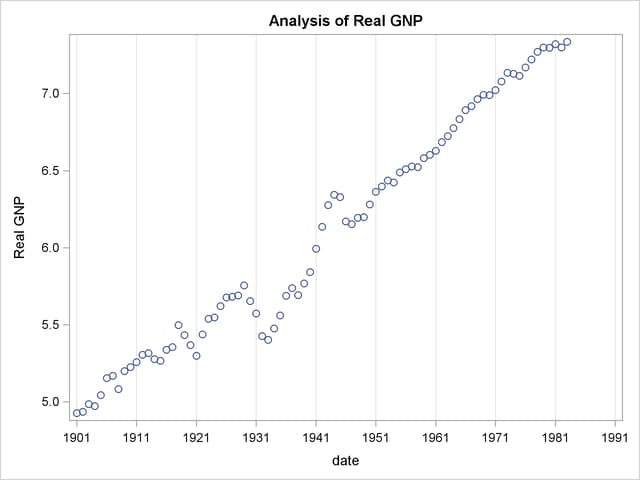The (linear) trend-stationary process is estimated using the following form: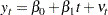where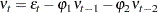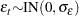The preceding trend-stationary model assumes that uncertainty over future horizons is bounded since the error term,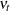, has a finite variance. The maximum likelihood AR estimates from the statements that follow are shown in Output 8.1.2:

```proc autoreg data=gnp;
model y = t / nlag=2 method=ml;
run;
```

Output 8.1.2 Estimating the Linear Trend Model
 Analysis of Real GNP

The AUTOREG Procedure

Maximum Likelihood Estimates
SSE 0.23954331 DFE 79
MSE 0.00303 Root MSE 0.05507
SBC -230.39355 AIC -240.06891
MAE 0.04016596 AICC -239.55609
MAPE 0.69458594 HQC -236.18189
Durbin-Watson 1.9935 Regress R-Square 0.8645
Total R-Square 0.9947

Parameter Estimates
Variable DF Estimate Standard Error t Value Approx
Pr > |t|
Variable Label
Intercept 1 4.8206 0.0661 72.88 <.0001
t 1 0.0302 0.001346 22.45 <.0001 Time Trend
AR1 1 -1.2041 0.1040 -11.58 <.0001
AR2 1 0.3748 0.1039 3.61 0.0005

Autoregressive parameters assumed given
Variable DF Estimate Standard Error t Value Approx
Pr > |t|
Variable Label
Intercept 1 4.8206 0.0661 72.88 <.0001
t 1 0.0302 0.001346 22.45 <.0001 Time Trend

Nelson and Plosser (1982) failed to reject the hypothesis that macroeconomic time series are nonstationary and have no tendency to return to a trend line. In this context, the simple random walk process can be used as an alternative process: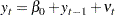where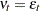and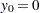. In general, the difference-stationary process is written as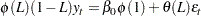where
is the lag operator. You can observe that the class of a difference-stationary process should have at least one unit root in the AR polynomial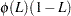.

The Dickey-Fuller procedure is used to test the null hypothesis that the series has a unit root in the AR polynomial. Consider the following equation for the augmented Dickey-Fuller test: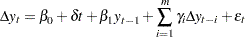where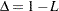. The test statistic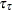is the usual t ratio for the parameter estimate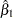, but thedoes not follow a t distribution.

The following code performs the augmented Dickey-Fuller test with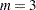and we are interesting in the test results in the linear time trend case since the previous plot reveals there is a linear trend.

```proc autoreg data = gnp;
model y = / stationarity =(adf =3);
run;
```

The augmented Dickey-Fuller test indicates that the output series may have a difference-stationary process. The statistic Tau with linear time trend has a value of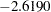and its p-value is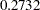. The statistic Rho has a p-value of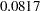which also indicates the null of unit root is accepted at the 5% level. (See Output 8.1.3.)

Output 8.1.3 Augmented Dickey-Fuller Test Results
 Analysis of Real GNP

The AUTOREG Procedure

Augmented Dickey-Fuller Unit Root Tests
Type Lags Rho Pr < Rho Tau Pr < Tau F Pr > F
Zero Mean 3 0.3827 0.7732 3.3342 0.9997
Single Mean 3 -0.1674 0.9465 -0.2046 0.9326 5.7521 0.0211
Trend 3 -18.0246 0.0817 -2.6190 0.2732 3.4472 0.4957

The AR(1) model for the differenced series DY is estimated using the maximum likelihood method for the period 1902 to 1983. The difference-stationary process is written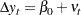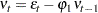The estimated value of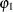is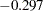and that of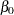is 0.0293. All estimated values are statistically significant. The PROC step follows:

```proc autoreg data=gnp;
model dy = / nlag=1 method=ml;
run;
```

The printed output produced by the PROC step is shown in Output 8.1.4.

Output 8.1.4 Estimating the Differenced Series with AR(1) Error
 Analysis of Real GNP

The AUTOREG Procedure

Maximum Likelihood Estimates
SSE 0.27107673 DFE 80
MSE 0.00339 Root MSE 0.05821
SBC -226.77848 AIC -231.59192
MAE 0.04333026 AICC -231.44002
MAPE 153.637587 HQC -229.65939
Durbin-Watson 1.9268 Regress R-Square 0.0000
Total R-Square 0.0900

Parameter Estimates
Variable DF Estimate Standard Error t Value Approx
Pr > |t|
Intercept 1 0.0293 0.009093 3.22 0.0018
AR1 1 -0.2967 0.1067 -2.78 0.0067

Autoregressive parameters assumed given
Variable DF Estimate Standard Error t Value Approx
Pr > |t|
Intercept 1 0.0293 0.009093 3.22 0.0018Previous Page | Next Page | Top of Page